Courses

# Algebra of Complex Numbers - Complex Analysis, CSIR-NET Mathematical Sciences Mathematics Notes | EduRev

## Mathematics : Algebra of Complex Numbers - Complex Analysis, CSIR-NET Mathematical Sciences Mathematics Notes | EduRev

The document Algebra of Complex Numbers - Complex Analysis, CSIR-NET Mathematical Sciences Mathematics Notes | EduRev is a part of the Mathematics Course Mathematics for IIT JAM, CSIR NET, UGC NET.
All you need of Mathematics at this link: Mathematics

At this point we have considered only real-valued functions of a real variable. That is, all of our work has centered on functions of the form f : R → R, functions which take a real number to a real number. In this chapter we will discuss complex numbers and the calculus of associated functions. In particular, if we let C represent the set of all complex numbers, then we will be interested in functions of the form f : R → C and f : C → C .
We will begin the story in this section with a discussion of what complex numbers are and how we work with them.

Perhaps because of their name, it is sometimes thought that complex numbers are in some way more mysterious than real numbers, that a number such as i =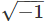is not as “real” as a number like 2 or −351.127 or even π. However, all of these numbers are equally meaningful, they are all useful mathematical abstractions. Although complex numbers are a relatively recent invention of mathematics, dating back just over 200 years in their current form, it is also the case that negative numbers, which were once called ﬁctitious numbers to indicate that they were less “real” than positive numbers, have only been accepted for about the same period of time, and we have only started to understand the nature of real numbers during the past 150 years or so. In fact, if you think about their underlying meaning, π is a far more “complex” number than i.

Although complex numbers originate with attempts to solve certain algebraic equations, such as we will give a geometric deﬁnition which identiﬁes complex numbers with points in the plane. This deﬁnition not only gives complex numbers a concrete geometrical meaning, but also provides us with a powerful algebraic tool for working with points in the plane.

x2 + 1 = 0,

Deﬁnition A complex number is an ordered pair of real numbers with addition deﬁned by

(a, b) + (c, d) = (a + c, b + d)                     (7.1.1)

and multiplication deﬁned by

(a, b) × (c, d) = (ac − bd, ad + bc),                  (7.1.2)

where a, b, c, and d are any real numbers.

We will let i denote the complex number (0, 1). Then, by our deﬁnition of multiplication,

i2 = (0, 1) × (0, 1) = (0 − 1, 0 + 0) = (−1, 0).                                      (7.1.3)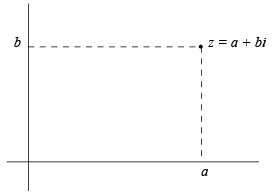Figure 7.1.1 Geometric representation of a complex number

If we identify the real number a with the complex number (a, 0), then we have

ai = (a, 0) × (0, 1) = (0 − 0, a + 0) = (0, a).

Then for any two real numbers, we have

(a, b) = (a, 0) + (0, b) = a + bi.                                 (7.1.4)

That is, a + bi is another way to write the complex number (a, b). In particular, with this convention, (7.1.3) becomes

i2 = −1,                                       (7.1.5)

that is,

i =(7.1.6)

Moreover, we may write (7.1.1) as

(a + bi) + (c + di) = (a + c) + (b + d)i                         (7.1.7)

and (7.1.2) as

(a + bi) × (c + di) = (ac − bd) + (ad + bc)i.                         (7.1.8)

In fact, we may view the latter as a consequence of the ordinary algebraic expansion of the product

(a + bi)(c + di)

combined with the equality i2 = −1. That is,

(a + bi)(c + di) = ac + adi + bci + bdi2 = (ac − bd) + (ad + bc)i.

It also follows from this formulation that if r is a real number, which we identify with r + 0i, and z = a + bi is a complex number, then

rz = r(a + bi) = (r + 0i)(a + bi) = ra + rbi.                 (7.1.9)

As indicated above, we let C denote the set of all complex numbers. Because of our identiﬁcation of C with the plane, we usually refer to C as the complex plane. Since the description of complex numbers as points in the plane is often associated with the work of Carl Friedrich Gauss (1777-1855) (although appearing ﬁrst in the work of Caspar Wessel (1745-1818)), C is also referred to as the Gaussian plane.

Example (3 + 4i) + (5 − 6i) = 8 − 2i.
Example (2 + i)(3 − 2i) = 6 − 4i + 3i − 2i2 = 8 − i.
Example −3(4 + 2i) = −12 − 6i.

We have yet to deﬁne subtraction and division for complex numbers. If z and w are complex numbers, we may deﬁne

z − w = z + (−1)w.                                          (7.1.10)

It follows that if z = a + bi and w = c + di, then

z − w = a + bi + (−c − di) = (a − c) + (b − d)i.                 (7.1.11)

As a ﬁrst step toward deﬁning division, note that if z = a + bi with either a ≠ 0 or b ≠ 0, then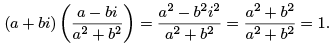In other words,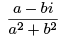is the multiplicative inverse, or reciprocal, of z = a + bi. Hence we will write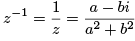(7.1.12)

Given another complex number w, we may deﬁne w divided by z by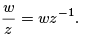(7.1.13)

Deﬁnition Given a complex number z = a + bi, the number a − bi is called the conjugate of z and is denoted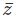.

Note that if z = a + bi, then

z= (a + bi)(a − bi) = a2 + b2 .                   (7.1.14)

Hence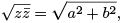(7.1.15)

which is the distance in the complex plane from z to the origin.

Deﬁnition Given a complex number z, the magnitude of z, denoted |z|, is deﬁned by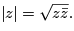(7.1.17)

Although (7.1.12) and (7.1.17) are useful expressions, in most situations the easiest way to simplify a quotient of two complex numbers is to multiply numerator and denominator by the conjugate of the denominator.

Example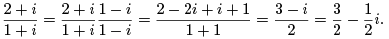Deﬁnition : Given a complex number z = a + bi, we call a the real part of z, denoted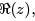, and b the imaginary part of z, denoted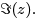Because of this deﬁnition, we call the horizontal axis of the complex plane the real axis and the vertical axis the imaginary axis. In this way we may identify the real number line with the the real axis of the complex plane. A complex number of the form bi, where b is a real number, lies on the imaginary axis of the complex plane and is said to be purely imaginary. However, we should be careful with our interpretation of this terminology: a purely imaginary number is just as “real”, in the ordinary sense of real, as a real number, in the same way that an irrational number is just as “rational”, in the sense of reasonable, as a rational number. Note that with this terminology, the conjugateof a complex number z is the point in the complex plane obtained by reﬂecting z about the real axis..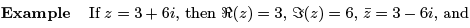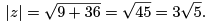With the above deﬁnitions, we may work with the arithmetic and algebra of complex numbers in the same way we work with real numbers. For example, for any complex numbers z and w,

z+w = w+z

and

zw = wz.

You will be asked to verify these and other standard properties of the complex numbers in Problem 7 at the end of this section.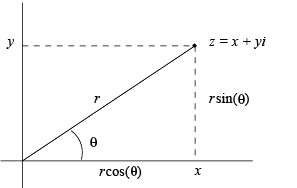Figure 7.1.2 Polar coordinates for a complex number

Polar notation

When we write a complex number z in the form z = x + yi, we refer to x and y as the rectangular or Cartesian coordinates of z. We now consider another method of representing complex numbers. Let us begin with a complex number z = x + yi written in rectangular form. Assume for the moment that x and y are not both 0. If we let θ be the angle between the real axis and the line segment from (0, 0) to (x, y), measured in the counterclockwise direction, then z is completely determined by the two numbers |z| and θ. We call θ the argument of z and denote it by arg(z). Geometrically, if we are given |z| and θ, we can locate z in the complex plane by taking the line segment of length |z| lying on the positive real axis, with a ﬁxed endpoint at the origin, and rotating it counterclockwise through an angle θ; the ﬁnal resting point of the rotating endpoint is the location of z . Algebraically, if z = x + yi is a complex number with r = |z| and θ = arg(z), then

x = r cos(θ)                          (7.1.18)

and

y = r sin(θ).                     (7.1.19)

Together, r and θ are called the polar coordinates of z. See Figure 7.1.2.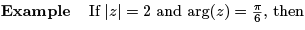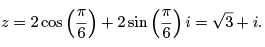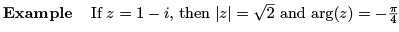Note that in the last example we could have taken arg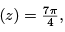or, in fact,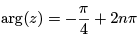for any integer n. In particular, there are an inﬁnite number of possible values for arg(z) and we will let arg(z) stand for any one of these values. At the same time, it is often important to choose arg(z) in a consistent fashion; to this end, we call the value of arg(z) which lies in the interval (−π, π] the principal value of arg(z) and denote it by Arg(z). For our example,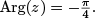In general, if we are given a complex number in rectangular coordinates, say z = x + yi, then, as we can see from Figure 7.1.2, the polar coordinates r = |z| and θ = Arg(z) are determined by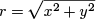(7.1.20)

and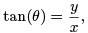(7.1.21)

where the latter holds only if x ≠ 0. If x = 0 and y ≠ 0, then z is purely imaginary and hence lies on the imaginary axis of the complex plane. In that case, θ = π/2  if y > 0 and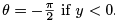. If both x = 0 and y = 0, then z is completely speciﬁed by the condition r = 0 and θ may take on any value.

Note that, since the range of the arc tangent function is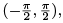the condition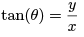only implies that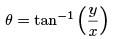if x > 0, that is, if θ is between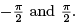Example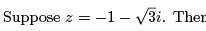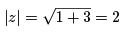and, if θ = Arg(z),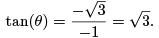Since z lies in the third quadrant, we have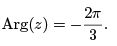Now suppose z1 and z2 are two nonzero complex numbers with |z1| = r1 , |z2| = r2 , arg(z1) = θ1 , and arg(z2) = θ2. Then

z1 = r1 cos(θ1) + r1 sin(θ1)i = r1 (cos(θ1) + sin(θ1)i)

and

z2 = r2 cos(θ2) + r2 sin(θ2)i = r2 (cos(θ2) + sin(θ2)i).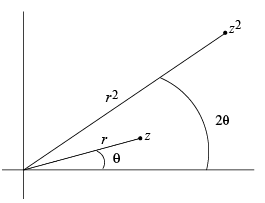Figure 7.1.3 Geometry of z and z2 in the complex plane

Hence

z1 z2 = (r1 cos(θ1) + r1 sin(θ1)i)(r2 cos(θ2) + r2 sin(θ2)i)
= r1 r2 (cos(θ1) cos(θ2) + cos(θ1) sin(θ2)i + sin(θ1) cos(θ2)i − sin(θ1) sin(θ2))
= r1 r2 (cos(θ1) cos(θ2) − sin(θ1) sin(θ2) + (sin(θ1) cos(θ2) + cos(θ1) sin(θ2))i)
= r1 r2 (cos(θ1 + θ2) + sin(θ1 + θ2)i).

It follows that

|z1 z2 | = r1 r2 = |z1 ||z2|                   (7.1.22)

and

arg(z1 z2) = θ1 + θ2 = arg(z1) + arg(z2).                  (7.1.23)

In other words, the magnitude of the product of two complex numbers is the product of their respective magnitudes and the argument of the product of two complex numbers is the sum of their respective arguments.

In particular, for any complex number z, |z2| = |z|2 and arg(z2) = 2 arg(z). More generally, for any positive integer n,

|zn| = |z|n                     (7.1.24)

and

arg(zn) = n arg(z).                    (7.1.25)

See Figure 7.1.3.

If z is a complex number with |z| = r and arg(z) = θ, then

z = r(cos(θ) + sin(θ)i)

and= r(cos(θ) − sin(θ)i) = r(cos(−θ) + sin(−θ)i).                (7.1.26)

Hence

|| = |z|                     (7.1.27)

and

arg() = − arg(z),                 (7.1.28)

in agreement with our previous observation thatis obtained from z by reﬂection about the real axis.

If z1 and z2 are two nonzero complex numbers with |z1| = r1 , |z2| = r2 , arg(z1) = θ1 , and arg(z2) = θ2 , then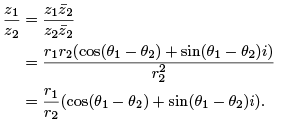Hence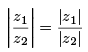(7.1.29)

and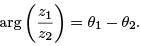(7.1.30)

In other words, the magnitude of the quotient of two complex numbers is the quotient of their respective magnitudes and the argument of the quotient of two complex numbers is the diﬀerence of their respective arguments.

Example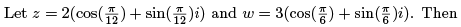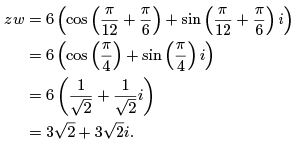Also,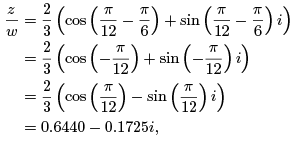where we have rounded the real and imaginary parts to four decimal places.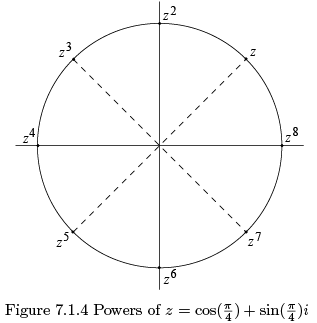Example Let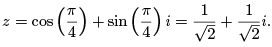Since |z| = 1 and arg(z) = π/4 , z is a point on the unit circle centered at the origin, one-eighth of the way around the circle from (1, 0) (see Figure 7.1.4). Then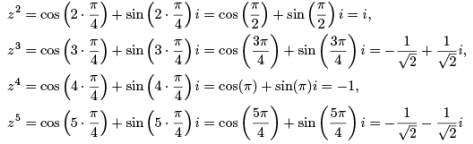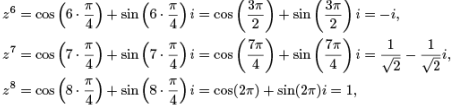and

z9 = zz8 = (z)(1) = z.

Hence each successive power of z is obtained by rotating the previous power through an angle of π/4 on the unit circle centered at the origin; after eight rotations, the point has returned to where it started. See Figure 7.1.4. Notice in particular that z is a root of the polynomial

P (w) = w8 − 1.

In fact, zn is a solution of w8 − 1 = 0 for any positive integer n since

(zn)8 − 1 = (z8)n − 1 = 1n − 1 = 1 − 1 = 0.

Thus there are eight distinct roots of P (w), namely, z , z2 , z3 , z4 , z5 , z6 , z7 , and z8 , only two of which, z4 = −1 and z8 = 1, are real numbers.

Offer running on EduRev: Apply code STAYHOME200 to get INR 200 off on our premium plan EduRev Infinity!

197 docs

,

,

,

,

,

,

,

,

,

,

,

,

,

,

,

,

,

,

,

,

,

,

,

,

;